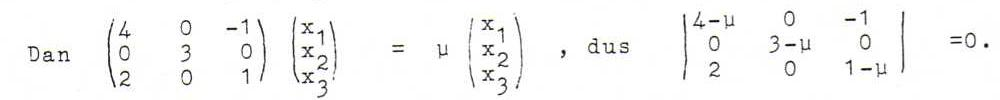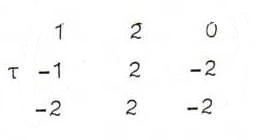### COURSE OF PROJECTIVE GEOMETRY

§ 16:

O63 Let λ(x1,x2,x3) be a fixed point (x0).We find μ=3 en x1 = x3 (axis) or μ=2 and (x1,x2,x3) = λ(1/2,0,1) (centre).
The matrix is non-singular because 0 is not an eigenvalue.

O64 Let A be a regular transformation of ℜ3 that induces φ. We construct the matrix of A on the natural base.
A(0,1,1) = σ(1,0,0), A(1,0,-1) = τ(1,1,0), A(0,1,0) = ρ(1,1,1), A(-2,2,3) = ω(1,0,1).
By sweeping we find
A(0,0,1) = (σ-ρ, -ρ, -ρ), A(1,0,0) = (τ+σ-ρ, τ-ρ, -ρ), A(0,1,0) = (ρ,ρ,ρ);
A(-2,2,3) = (ρ+σ-2τ, ρ-2τ, ρ) = (ω, 0, ω).
We find ρ = σ = 2τ and the matrix becomes